# Hierarchical clustering: how it works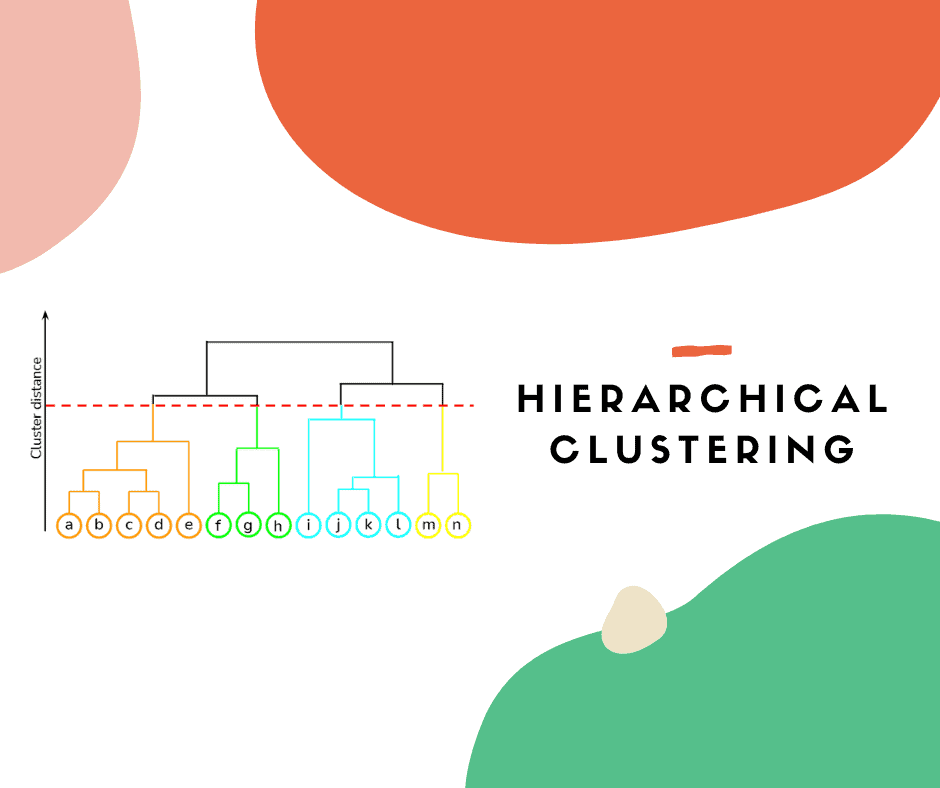Clustering algorithms allow data to be grouped according to their inherent characteristics. There are many algorithms that have been developed over the years. Hierarchical clustering, thanks to a graphical representation called a dendogram, makes it possible to visualize at a glance the composition of clusters and interpret their characteristics. Let us find out, step by step, how it works and how to interpret the results obtained.

## Share

Clustering algorithms make it possible to group unlabeled data into groups that share characteristics. There are several methods, and we explained some of them in the articles DBSCAN: how it works and K-Means: how it works. In this article, however, we will discuss hierarchical clustering. This methodology allows us to create a hierarchy between data points that can then be used to define groups (i.e., clusters). Let’s find out how it works step by step.

Suppose a store has collected the age of 9 customers, labeled C1-C9, and the amount spent by each of them in the store in the past month.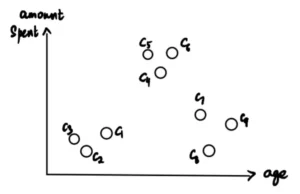Now the store wants to identify segments or clusters of its customers to better understand their needs. To do this, data scientists (i.e., we), are in charge of analyzing the data. In this context, having even little data, we will use hierarchical clustering.

## Creating clusters and dendrograms

We start by making each individual data point a cluster. Nine clusters are thus formed: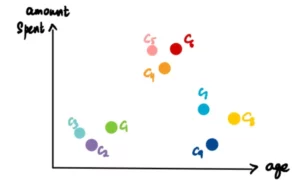We take the two closest clusters (for distance measurement we refer you to the Distances section) and make them one cluster. Since C2 and C3 are the closest, they form one cluster. This gives a total of 8 clusters.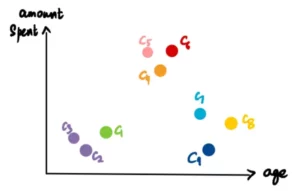We now represent this cluster with a graph called a Dendrogram. The x-axis represents the points (in our case the customers) and the y-axis represents the distance between the clusters.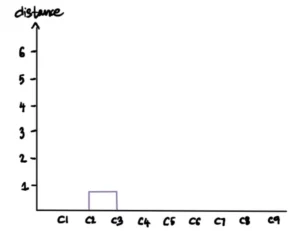We repeat the previous operation. We take the two closest clusters (C5 and C6), transform them into a single cluster and plot it on the dendrogram.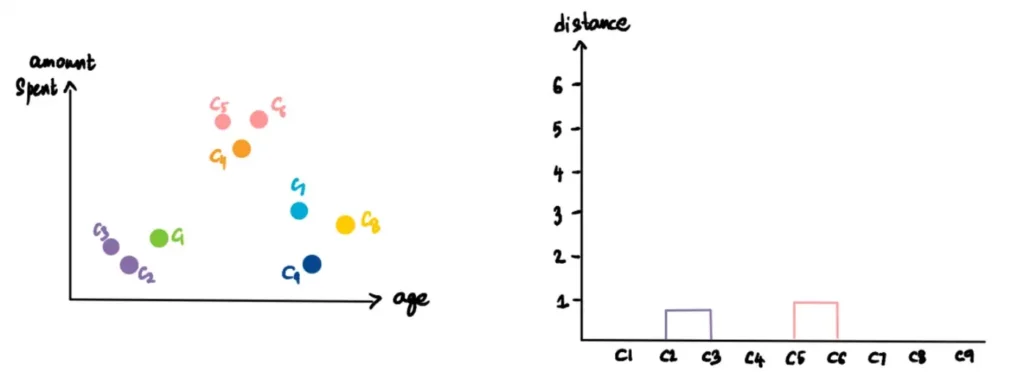We continue repeating this process until only one cluster remains.

Here is how to create the dendrogram and hierarchical clusters.

## Distances

Previously, we talked about finding the closest clusters, but how exactly do we measure this closeness?

Suppose we have the pink, blue and purple clusters. Now we want to figure out whether we should group the pink cluster with the blue cluster or with the purple cluster.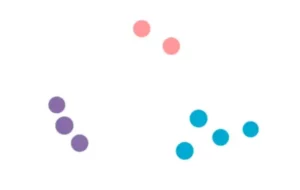There are four ways to do this. But before we discuss them, let’s briefly talk about distance. In this context, when I talk about distance I mean Euclidean distance. So if p with coordinates (p₁, p₂,…, pₙ) and q with coordinates (q₁, q₂,…, qₙ) are two points in n-dimensions, the Euclidean distance between them is:

$$d(p,q)=d(q,p) = \sqrt{(q_1+p_1)^2+ (q_2+p_2)^2 + ... + (q_n+p_n)^2} = \sqrt{\sum_{i=1}^N(q_i+p_i)^2 }$$

In our case, since the data are two-dimensional, the Euclidean distance between two points will be: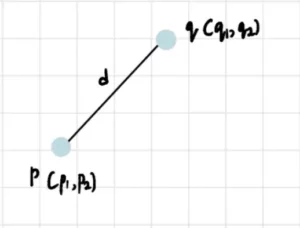Of course, other distances can be used as discussed in the K-Means article. To give you a quick indication, the most commonly used distances, besides Euclidean, are Manhattan, Minkowski and cosine distances.

Now that we understand how to interpret distances, the 4 ways to determine proximity are:

Single-Linkage: in the single-linkage method, we define distance as the shortest distance between two points in each cluster. Therefore, we compare the distance between the nearest points in the pink and purple clusters with the distance between the nearest points in the blue and pink clusters. The smaller of the two distances determines the closest cluster to the pink cluster.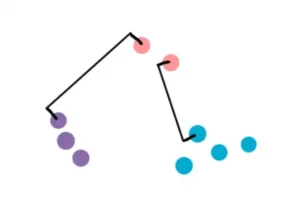Complete-Linkage: is similar to Single-Linkage, but in this case the distance is measured between the farthest pair of points in two clusters.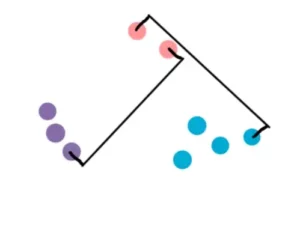Average Linkage: as the name suggests, the distance is defined as the average distance between each point in the first cluster and each point in the second cluster. The mathematical formula for calculating the distance is as follows:

$$L(r,s) = \frac{1}{n_rn_s}\sum_{i=1}^{n_r} \sum_{j=1}^{n_s} D(x_{ri}, x_{sj})$$

Don’t be scared! The important thing is that you understand the idea behind the method.

Centroid method: this involves finding the centroid of each cluster and then finding the distance between them. For the centroid calculation I refer you to the article on K-means.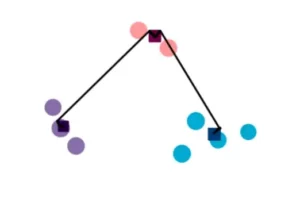### Cluster customization

We know that our final dendrogram looks like this: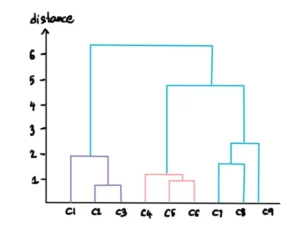We can customize the number of clusters we want by specifying a threshold for distance. For example, suppose we do not want clusters with distances greater than 3. Then we draw a line for the threshold of 3: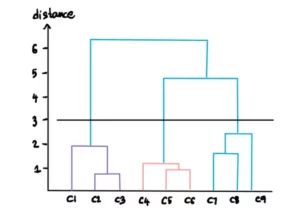This line implies that beyond this level the clusters will not be considered. Therefore, only the 3 clusters below the threshold line remain: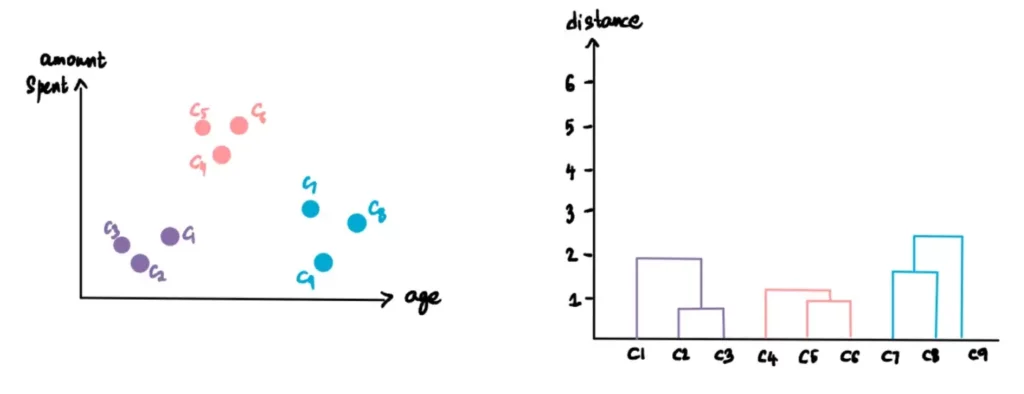If we set the distance threshold at 5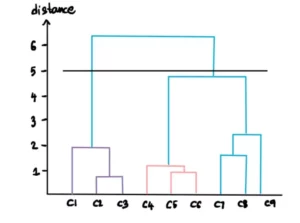then we will get only 2 clusters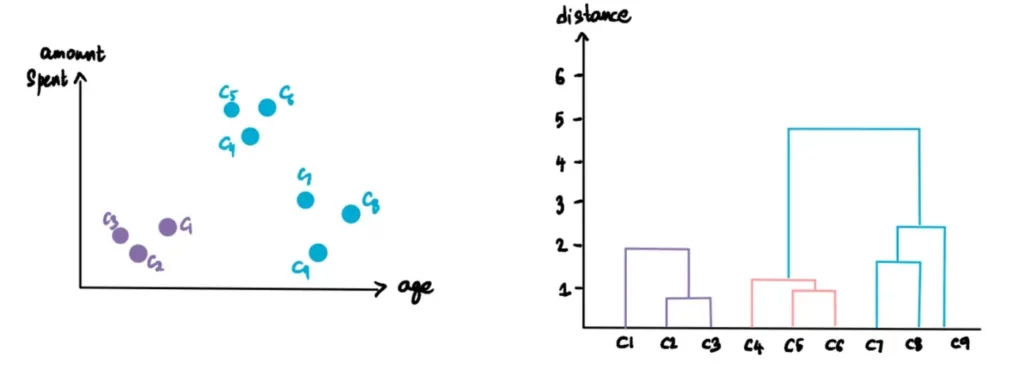### Optimal number of clusters

The optimal number of clusters is obtained by finding the longest line that does not cross any horizontal line (threshold). In our case, this will be the longest line: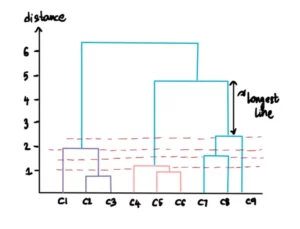We must then draw a horizontal line across this maximum distance line, and this will be the threshold for calculating the clusters. The result will be as follows: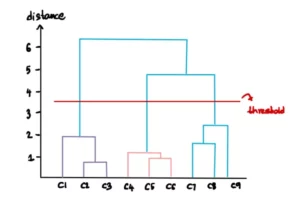### How do we use the obtained clusters?

Now that we have finished the clustering process and found that there are 3 optimal clusters, what inferences can the store draw from this?

In our example, we assume that the common characteristics of the points belonging to each cluster determine 3 categories: younger customers who do not spend much, older customers who spend less, and the average segment that spends a lot. The store can now use this information to its advantage. It can optimize its business by amplifying the services, products and offers offered to the third segment of middle-aged customers. It can pool its resources to make this group happier: offer them more choices that suit their tastes, carry the latest products, or even offer them exclusive shopping hours.

This is a simple example of how clustering can be used, but the possibilities are endless!

## More To ExploreDBMS

### SQL: correlation

In the SQL language, it is possible to condition the execution of a query on the values of the tuples being parsed. This behavior is defined by correlation, that is, conditions in the WHERE clause of a nested query that relate to attributes of the tables in the outer query. Through some examples we will discover which operators use this construct, how to appropriately define correlations, and its alternatives.DBMS

### SQL: Common Table Expression

Expressing complex queries in SQL language can be really difficult. Dividing the problem into mini-queries is the best strategy to get the desired results quickly without running into errors. Common Table Expressions, also called CTEs, allow the queries of our interest to be defined once to be reused multiple times when needed. Their clean syntax increases the readability of SQL scripts, allows us to debug written code and to create very complex logic to filter and extract data. Through some examples we will discover their potential.

### One Response